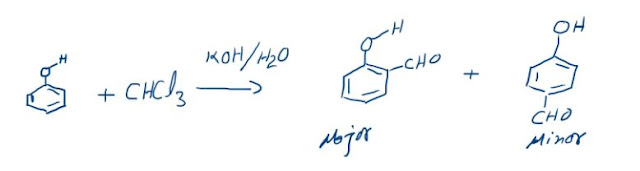## COMBINED GAS LAW

The laws that we have studied so far can be combined to obtain a universal law and equation describing it. The individual laws are bound by constraints but the overall law doesn’t have any constraints.
The individual laws are:
P α 1/V (at constant T and n)
V α T (at constant P and n)
P α T (at constant V and n)
V α n (at constant P and T)
Looking at the trend above, we can say, on removing the constraints:
PV α n T
Or
PV / n T = constant
This constant is called Universal Gas constant represented by “R”.
Therefore we obtain the following equation:
PV = n R T
This is called “Ideal Gas Equation” and is one of the most important equations in Physical Chemistry. It relates all the state variables for an ideal gas. Through this equation we can understand the behavior of ideal gases in various systems. But first we will analyse the constant R and its units which are very important.

R = PV/ n T
SI units: Nm/mol-K or J/mol-K and its value in these units is 8.314 J/mol-K
The other common unit used is: L-atm/mol-K and its value is 0.0821 L-atm/mol-K
Various other units are also in use like 1.98 Cal /mol-K but in this chapter we will be mostly dealing with the first two equations. We have to remember that whenever we take SI units of pressure and volume that is N/m2 and m3 then we have to take R as 8.314 J/mol-K and whenever we take pressure in atmosphere and volume in L then we have to take R as 0.0821 L-atm/mol-K. Always try not to use any other set of units or else it can be highly misleading. R is called universal constant because it is independent of any other parameter.
The ideal gas equation can also represented like this:
PV/ n T = constant
P1V1/n1T1 = P2V2/n2T2
Any two states of ideal gas can be related by the above relationship.
APPLICATIONS OF THE IDEAL GAS EQUATION
The equation seems to be straight forward and simple but it has numerous applications. To understand these applications we must focus on constants and variables in the equation.
If any system needs to be analysed then follow the following steps:

§  Identify the system; it may be a vessel, gas etc.
§  Identify the variables and constants (out of the state variables P, V, T and n) after identifying the process involved
§  Take the variables on one side of the equation and the constants on the other
§  We will obtain the equation of state for the given problem
§  Apply it on the given states and carry out the calculations.

### The Reimer-Tiemann reaction: Reaction and its Mechanism

Reimer-Tiemann Reaction Phenols on reaction with chloroform in the presence of sodium hydroxide (or potassium hydroxide) solution give hydro...